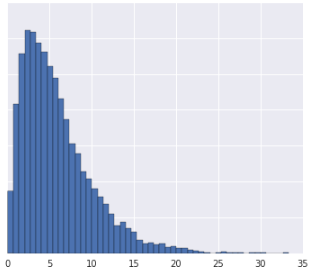0%

## 规范化数据 Normalizing Data

$$\begin{array}{*{20}{c}} {x' = (x - \mu )/\sigma } \\ {\mu = {\text{mean}}} \\ {\sigma = {\text{standard deviation}}} \end{array}$$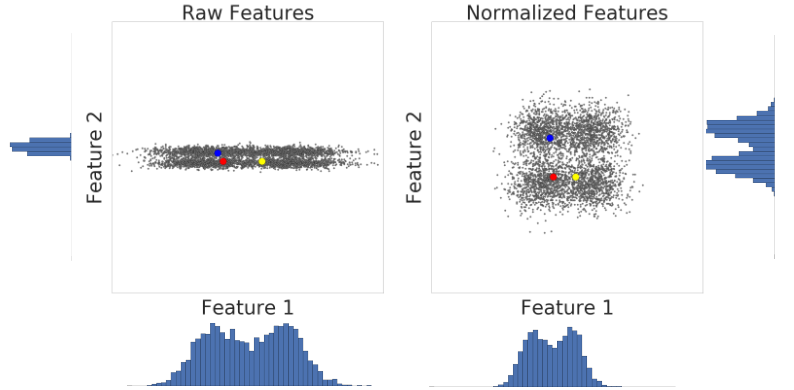1. 数据服从高斯分布
2. 缺少足够的数据来创建分位数

## Log转换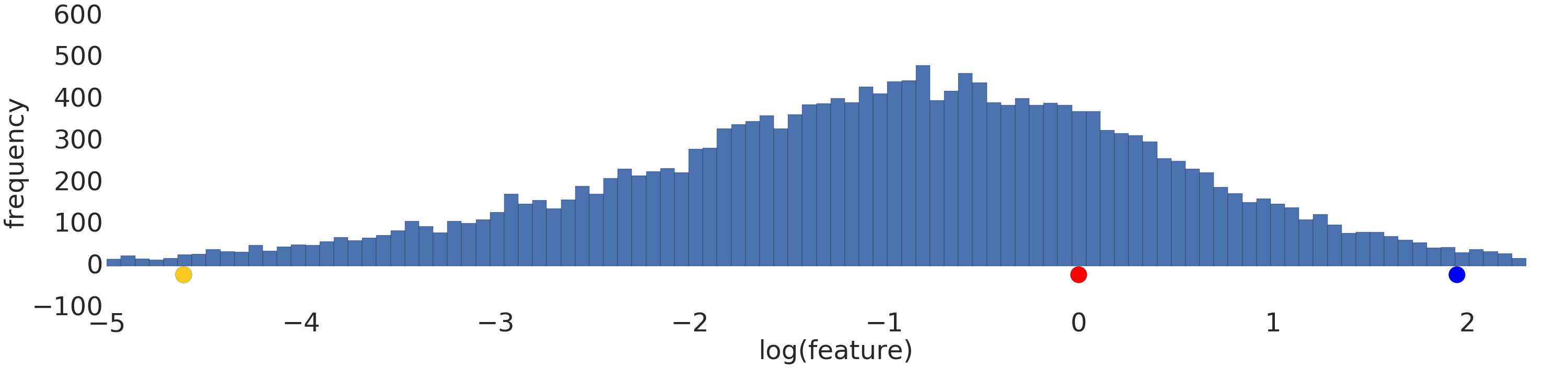## 分位数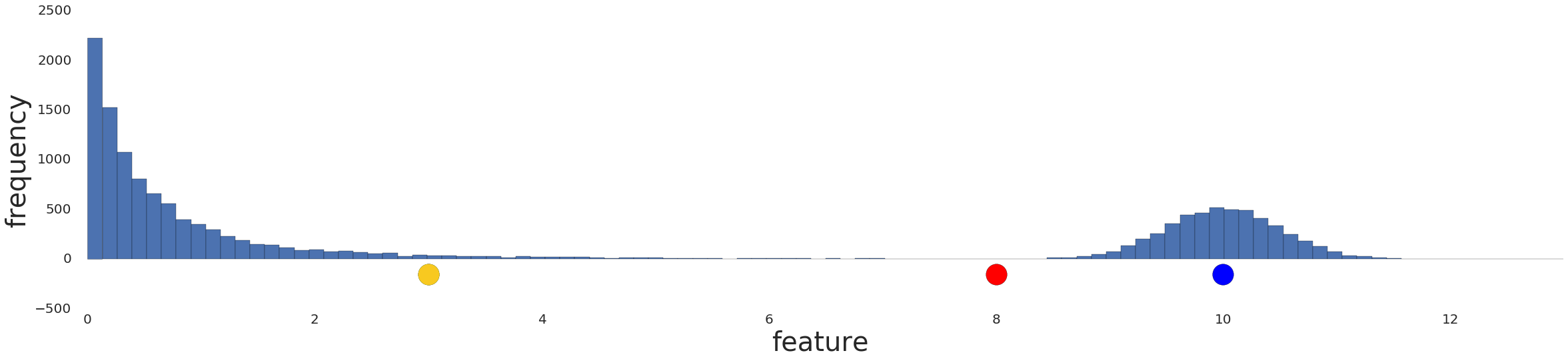1. 确定间隔的数量。
2. 定义区间，使每个区间具有相同数量的样本。
3. 用样本所在区间的索引替代原先的样本值。
4. 将索引值缩放到[0,1]，使索引与其他特征的数据范围相同。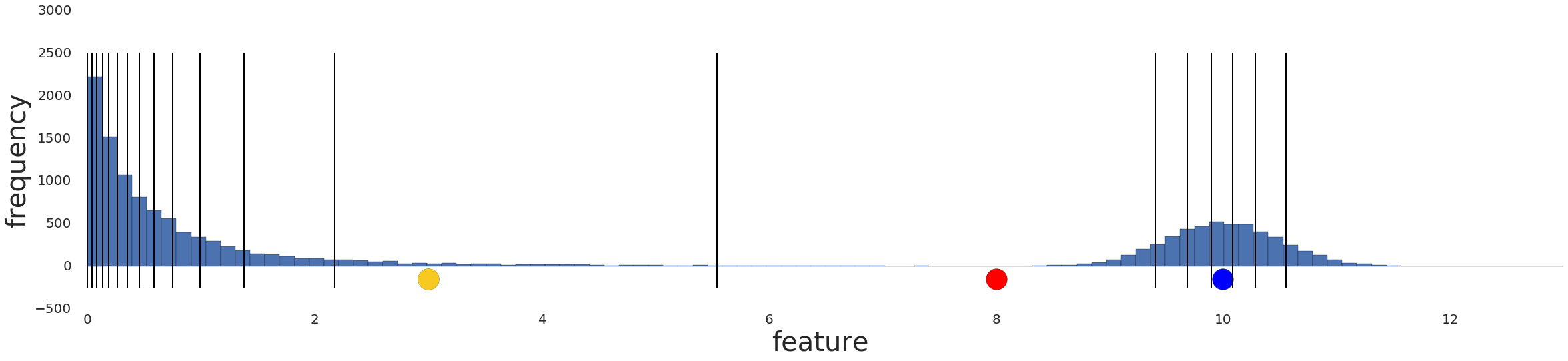$$sim(A,B) \approx 1 - |{\text{prob}}[x > A] - {\text{prob}}[x > B]|$$ $$sim(A,B) \approx 1 - |{\text{quantile}}(A) - {\text{quantile}}(B)|$$